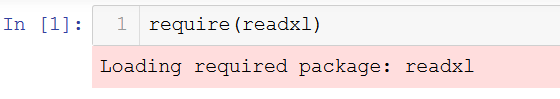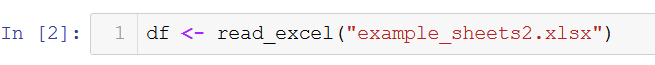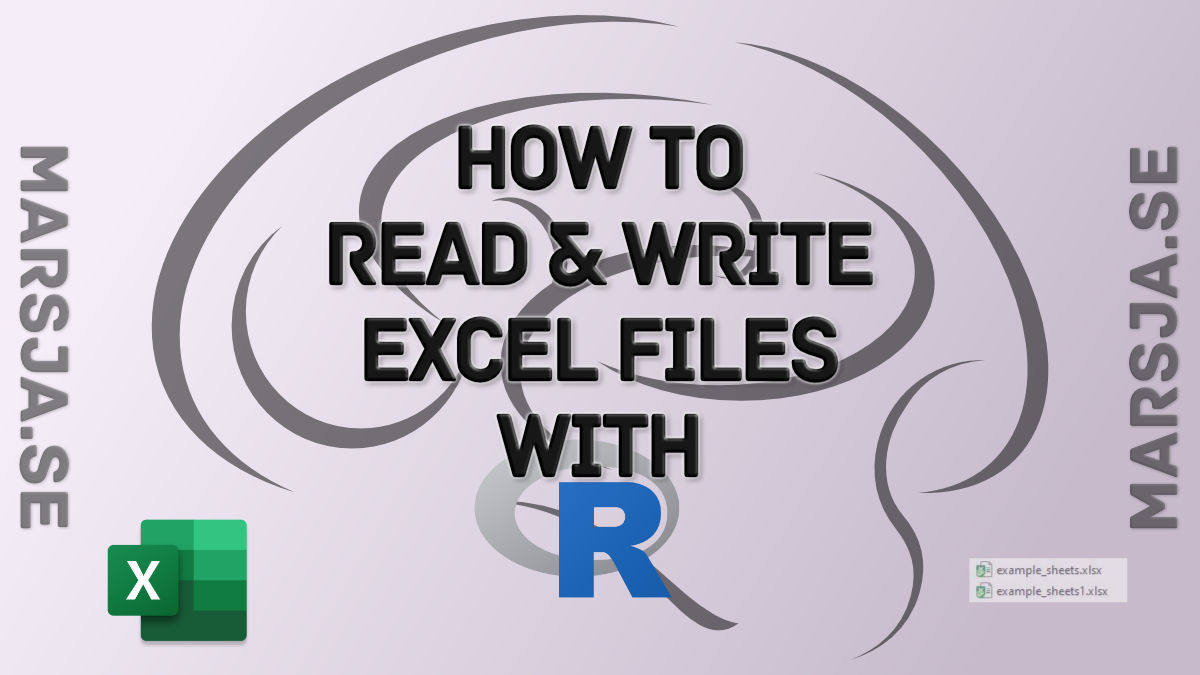8 Shares

In this tutorial, we will learn how to work with Excel files in R statistical programming environment. It will provide an overview of how to use R to load xlsx files and write spreadsheets to Excel.

In the first section, we will go through, with examples, how to use R read an Excel file. More specifically, we are going to learn how to;

• import multiple spreadsheets and combine them to one dataframe,
• import Excel datasets using RStudio

Furthermore, in the last part we are going to focus on how to export dataframes to Excel files. More specifically, we are going to learn how to write;

• Excel files, rename the sheet
• to multiple sheets,
• multiple dataframes to a Excel file

## How to Install R Packages

Now, before we continue with this Excel in R tutorial we are going to learn how to install the needed packages. In this post, we are going to use tidyverses readxl and the xlsx package to read xlsx files to dataframes.

Note, we are mainly using xlsx, in this post, because readxl cannot write Excel files, only import them into R.

```.wp-block-code {
border: 0;
}

.wp-block-code > div {
overflow: auto;
}

.shcb-language {
border: 0;
clip: rect(1px, 1px, 1px, 1px);
-webkit-clip-path: inset(50%);
clip-path: inset(50%);
height: 1px;
margin: -1px;
overflow: hidden;
position: absolute;
width: 1px;
word-wrap: normal;
word-break: normal;
}

.hljs {
box-sizing: border-box;
}

.hljs.shcb-code-table {
display: table;
width: 100%;
}

.hljs.shcb-code-table > .shcb-loc {
color: inherit;
display: table-row;
width: 100%;
}

.hljs.shcb-code-table .shcb-loc > span {
display: table-cell;
}

.wp-block-code code.hljs:not(.shcb-wrap-lines) {
white-space: pre;
}

.wp-block-code code.hljs.shcb-wrap-lines {
white-space: pre-wrap;
}

.hljs.shcb-line-numbers {
border-spacing: 0;
counter-reset: line;
}

.hljs.shcb-line-numbers > .shcb-loc {
counter-increment: line;
}

.hljs.shcb-line-numbers .shcb-loc > span {
}

.hljs.shcb-line-numbers .shcb-loc::before {
border-right: 1px solid #ddd;
content: counter(line);
display: table-cell;
text-align: right;
-webkit-user-select: none;
-moz-user-select: none;
-ms-user-select: none;
user-select: none;
white-space: nowrap;
width: 1%;
}
```# Install tidyverse
install.packages("tidyverse")

# how to install xlsx
install.packages("xlsx")```Code language: R (r)```

Now, Tidyverse comes with a lot of useful packages. For example, using the package dplyr (part of Tidyverse) you can remove duplicates in R, and rename a column in R’s dataframe.

### How to install RStudio

In the final example, we are going to read xlsx files in R using the interactive development environment RStudio. Now, RStudio is quite easy to install. In this post, we will cover two methods for installing RStudio.

Here’s two steps for installing RStudio:

2. Click on the installation file and follow the instructions

Now, there’s another option to get both R statistical programming environment and the great general-purpose language of Python. That is, to install the Anaconda Python distribution.

Note, RStudio is a great Integrated Development Environment for carrying out data visualization and analysis using R. RStudio is mainly for R but we can also use other programming languages ( e.g., Python). That is, we typically don’t use RStudio for importing xlsx files only.

## How to Read Excel Files to R Dataframes

Can R read xlsx files? In this section, we are going to find out that the answer is, of course, “yes”. We are going to learn how to load Excel files using Tidyverse (e.g., readxl).

More specifically, in this section, we are going to learn how to read Excel files and spreadsheets to dataframes in R. In the read Excel examples we will read xlsx files from both the hard drive and URLs.

### How to Import an Excel file in R using read_excel

First, we are going to load the r-package(s) we need. How do I load a package in R? It can be done either by using the library or require functions. In the next code chunk, we are going to load readxl so we can use the read_excel function to read Excel files into R dataframes.

``require(readxl)`Code language: R (r)`

If we look at the documentation for the function, read_excel, that we are going to use in this tutorial we can see that it takes a range of arguments.

Now it’s time to learn how to use read_excel to read in data from an Excel file. The easiest way to use this method is to pass the file name as a character. If we don’t pass any other parameters, such as sheet name, it will read the first sheet in the index. In the first example we are not going to use any parameters:

``````df <- read_excel("example_sheets2.xlsx")

Here, the read_excel function reads the data from the Excel file into a tibble object. We can if we want to, change this tibble to a dataframe.

``df <- as.data.frame(df)`Code language: R (r)`

Now, after importing the data from the Excel file you can carry on with data manipulation if needed. It is, for instance, possible to remove a column, by name and index, with the R-package dplyr. Furthermore, if you installed tidyverse you will have a lot of tools that enable you to do descriptive statistics in R, and create scatter plots with ggplot2.

### Importing an Excel File to R in Two Easy Steps:

Time needed: 1 minute.

Here’s a quick answer to the question how do I import Excel data into R?? Importing an Excel file into an R dataframe only requires two steps, given that we know the path, or URL, to the Excel file:2. Import the XLSX file

Second, you can use read_excel function to load the .xlsx (or .xls) fileWe now know how to easily load an Excel file in R and can continue with learning more about the read_excel function.

In this section, we are going to learn how to read specific columns from an Excel file using R. Note, here we will also use the read.xlsx function from the package xlsx.

In this section, we are going to learn how to read certain columns from an Excel sheet using R. Reading only some columns from an Excel sheet may be good if we, for instance, have large xlsx files and we don’t want to read all columns in the Excel file. When using readxl and the read_excel function we will use the range parameter together with cell_cols.

When using read.xlsx, to import Excel in R, we can use the parameter colIndex to select specific columns from the sheet. For example, if want to create a dataframe with the columns PlayerSalary, and Position, we can accomplish this by adding 1, 3, and 4 in a vector:

``````require(xlsx)
cols <-  c(1, 2, 3)

sheetName='MLBPlayerSalaries', colIndex=cols)

### Handling Missing Data when we Import Excel File(s) in R

If someone has coded the data and used some kind of value to represent missing values in our dataset, we need to tell r, and the read_excel function, what these values are. In the next, R read Excel example, we are going to use the na parameter of the read_excel function. Here “-99” is what is codes as missing values.

#### Read Excel Example with Missing Data

In the example below, we are using the parameter na and we are putting in a character (i.e., “-99”):

``````df <- read_excel('SimData/example_sheets2.xlsx', 'Session2',
na = '-99')

The example datasets we’ve used in the how to use R to read Excel files tutorial can be found here and here.

### How to Skip Rows when Importing an xlsx File in R

In this section, we will learn how to skip rows when loading an Excel file into R. Here’s a link to the example xlsx file.

In the following, read xlsx in R examples we are going to use both read_excel and read.xlsx to read a specific sheet. Furthermore, we are also going to skip the first 2 rows in the Excel file.

Here, we will use the parameter sheet and put the characters ‘Session1’ to read the sheet named ‘Session1’. In a previous example, we just added the character ‘Session2’ to read that sheet.

Note, the first sheet will be read if we don’t use the sheet_name parameter. In this example, the important part is the parameter skiprow=2. We use this to skip the first two rows:

``````df <- read_excel('SimData/example_sheets.xlsx',
sheet='Session1', skip = 2)

#### How to Skip Rows when Reading Excel Files in R using read.xlsx

When working with read.xlsx we use the startRow parameter to skip the first 2 rows in the Excel sheet.

``````df <- read.xlsx('SimData/example_sheets.xlsx',
sheetName='Session1', startRow=3)```Code language: HTML, XML (xml)```

## Reading Multiple Excel Sheets in R

In this section of the R read excel tutorial, we are going to learn how to read multiple sheets into R dataframes.

There are two sheets: ‘Session1’, and ‘Session2, in the example xlsx file (example_sheets2.xlsx). In this file, each sheet has data from two experimental sessions.

We are now learning how to read multiple sheets using readxl. More specifically, we are going to read the sheets ‘Session1’ and ‘Session2’. First, we are going to use the function excel_sheets to print the sheet names:

``````xlsx_data <- "SimData/example_sheets.xlsx"

excel_sheets(path = xlsx_data)```Code language: R (r)```

Now if we want to read all the existing sheets in an Excel document we create a variable, called sheet_names.

After we have created this variable we use the lapply function and loop through the list of sheets, use the read_excel function, and end up with the list of dataframes (excel_sheets):

``````sheet_names <- excel_sheets(path = xlsx_data)

excel_sheets <- lapply(sheet_names , function(x) read_excel(path = xlsx_data, sheet = x))

str(excel_sheets)```Code language: R (r)```

When working with Pandas read_excel w may want to join the data from all sheets (in this case sessions). Merging Pandas dataframes are quite easy. We just use the concat function and loop over the keys (i.e., sheets):

``````df <- do.call("rbind", excel_sheets)

Again, there might be other tasks that we need to carry out. For instance, we can also create dummy variables in R.

# Reading Many Excel Files in R

In this section of the R read excel tutorial, we will learn how to load many files into an R dataframe.

For example, in some cases, we may have a bunch of Excel files containing data from different experiments or experimental sessions. In the next example, we are going to work with read_excel, again, together with the lapply function.

However, this time we just have a character vector with the file names and then we also use the paste0 function to paste the subfolder where the files are.

``````xlsx_files <- c("example_concat.xlsx",
"example_concat1.xlsx",
"example_concat3.xlsx")

dataframes &lt;- lapply(xlsx_files, function(x)
read_excel(path = paste0("simData/", x)))```Code language: R (r)```

Finally, we use the do.call function, again, to bind the dataframes together to one. Note, if we want, we can also use, the bind_cols function from the r-package dplyr (part of tidyverse).

``````df <- do.call("rbind", dataframes)

tail(df)```Code language: R (r)```

Note, if we want, we can also use, the bind_cols function from the r-package dplyr (part of tidyverse).

``dplyr::bind_rows(dataframes)`Code language: R (r)`

#### Reading all Files in a Directory in R

In this section, we are going to learn how to read all xlsx files in a directory. Knowing this may come in handy if we store every xlsx file in a folder and don’t want to create a character vector, like above, by hand. In the next example, we are going to use R’s Sys.glob function to get a character vector of all Excel files.

``xlsx_files <- Sys.glob('./simData/*.xlsx')`Code language: R (r)`

After we have a character vector with all the file names that we want to import to R, we just use lapply and do.call (see previous code chunks).

## Setting the Data type for data or columns

We can also, if we like, set the data type for the columns. Let’s use Pandas to read the example_sheets1.xlsx again. In the Pandas read_excel example below we use the dtype parameter to set the data type of some of the columns.

``````df <- read_excel('SimData/example_sheets2.xlsx',
col_types=c("text", "text", "numeric",
"numeric", "text"),
sheet='Session1')

str(df)```Code language: R (r)```

## Importing Excel Files in RStudio

Before we continue this Excel in R tutorial, we are going to learn how to load xlsx files to R using RStudio. This is quite simple, open up RStudio, click on the Environment tab (right in the IDE), and then Import Dataset. That is, in this section, we will answer the question of how do I import an Excel file into RStudio?

Now we’ll get a dropdown menu and we can choose from different types of sources. As we are going to work with Excel files we choose “From Excel…”:

In the next step, we klick “Browse” and go to the folder where our Excel data is located.

Now we get some alternatives. For instance, we can change the name of the dataframe to “df”, if we want (see image below). Furthermore, before we import the Excel file in RStudio we can also specify how the missing values are coded as well as rows to skip.

Finally, when we have set everything as we want we can hit the Import button in RStudio to read the datafile.

## Writing R Dataframes to Excel

Excel files can, of course, be created in R. In this section, we will learn how to write an Excel file using R. As for now, we have to use the r-package xlsx to write .xlsx files. More specifically, to write to an Excel file we will use the write.xlsx function:

We will start by creating a dataframe with some variables.

``````df <- data.frame("Age" = c(21, 22, 20, 19, 18, 23), "Names" = c("Andreas", "George", "Steve",
"Sarah", "Joanna", "Hanna"))

str(df)```Code language: R (r)```

Now that we have a dataframe to write to xlsx we start by using the write.xlsx function from the xlsx package.

``````library(xlsx)
write.xlsx(df, 'names_ages.xlsx',
sheetName = "Sheet1"```Code language: R (r)```

In the output below the effect of not using any parameters is evident. If we don’t use the parameter sheetName we get the default sheet name, ‘Sheet1’.

As can be noted in the image below, the Excel file has column (‘A’) containing numbers. These are the index from the dataframe.

In the next example we are going to give the sheet another name and we will set the row.names parameter to FALSE.

``````write.xlsx(df, 'names_ages.xlsx',
sheetName = "Names and Ages",
row.names=FALSE)```Code language: R (r)```

As can be seen, in the image above, we get a new sheet name and we don’t have the indexes as a column in the Excel sheet. Note, if you get the error ‘could not find function “write.xlsx”‘ it may be that you did not load the xlsx library.

### Writing Multiple Pandas Dataframes to an Excel File:

In this section, we are going to learn how to write multiple dataframes to one Excel file. More specifically, we will use R and the xlsx package to write many dataframes to multiple sheets in an Excel file.

First, we start by creating three dataframes and add them to a list.

``````df1 <-data.frame('Names' = c('Andreas', 'George', 'Steve',
'Sarah', 'Joanna', 'Hanna'),
'Age' = c(21, 22, 20, 19, 18, 23))

df2 <- data.frame('Names' =  c('Pete', 'Jordan', 'Gustaf',
'Sophie', 'Sally', 'Simone'),
'Age' = c(22, 21, 19, 19, 29, 21))

df3 <- data.frame('Names' = c('Ulrich', 'Donald', 'Jon',
'Jessica', 'Elisabeth', 'Diana'),
'Age' = c(21, 21, 20, 19, 19, 22))

dfs &lt;- list(df1, df2, df3)```Code language: R (r)```

Next, we are going to create a workbook using the createWorkbook function.

``wb <- createWorkbook(type="xlsx")`Code language: R (r)`

Finally, we are going to write a custom function that we are going to use together with the lapply function, later. In the code chunk below,

``````add_dataframes <- function(i){

df = dfs[i]
sheet_name = paste0("Sheet", i)
sheet = createSheet(wb, sheet_name)

}```Code language: R (r)```

It’s time to use the lapply function with our custom R function. On the second row, in the code chunk below, we are writing the workbook to an xlsx file using the saveWorkbook function:

``lapply(seq_along(dfs), function(x) multiple_dataframe(x))saveWorkbook(wb, 'multiple_Sheets.xlsx')`Code language: R (r)`

## Summary: How to Work With Excel Files in R

In this working with Excel in R tutorial we have learned how to:

• Load Excel files to dataframes:
• Import Excel sheets and skip rows
• Merging many sheets to a dataframe
• Reading many Excel files into one dataframe
• Write a dataframe to an Excel file
• Creating many dataframes and writing them to an Excel file with many sheets8 Shares